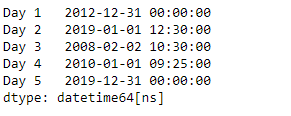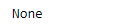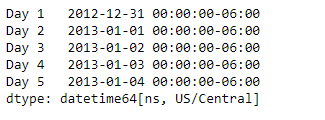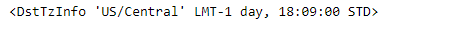# Python | Pandas Series.dt.tz

`Series.dt` can be used to access the values of the series as datetimelike and return several properties. Pandas` Series.dt.tz` attribute return the timezone if any, else it return None.

Syntax: Series.dt.tz

Parameter : None

Returns : timezone

Example #1: Use `Series.dt.tz` attribute to find the timezone of the underlying datetime based data in the given series object.

 `# importing pandas as pd ` `import` `pandas as pd ` ` `  `# Creating the Series ` `sr ``=` `pd.Series([``'2012-12-31'``, ``'2019-1-1 12:30'``, ``'2008-02-2 10:30'``, ` `               ``'2010-1-1 09:25'``, ``'2019-12-31 00:00'``]) ` ` `  `# Creating the index ` `idx ``=` `[``'Day 1'``, ``'Day 2'``, ``'Day 3'``, ``'Day 4'``, ``'Day 5'``] ` ` `  `# set the index ` `sr.index ``=` `idx ` ` `  `# Convert the underlying data to datetime  ` `sr ``=` `pd.to_datetime(sr) ` ` `  `# Print the series ` `print``(sr) `

Output :Now we will use `Series.dt.tz` attribute to find the timezone of the datetime data in the given series object.

 `# find the timezone ` `result ``=` `sr.dt.tz ` ` `  `# print the result ` `print``(result) `

Output :As we can see in the output, the `Series.dt.tz` attribute has returned `None` indicating the timezone for the given datetime data is not known.

Example #2 : Use `Series.dt.tz` attribute to find the timezone of the underlying datetime based data in the given series object.

 `# importing pandas as pd ` `import` `pandas as pd ` ` `  `# Creating the Series ` `sr ``=` `pd.Series(pd.date_range(``'2012-12-31 00:00'``, periods ``=` `5``, freq ``=` `'D'``, ` `                            ``tz ``=` `'US / Central'``)) ` ` `  `# Creating the index ` `idx ``=` `[``'Day 1'``, ``'Day 2'``, ``'Day 3'``, ``'Day 4'``, ``'Day 5'``] ` ` `  `# set the index ` `sr.index ``=` `idx ` ` `  `# Print the series ` `print``(sr) `

Output :Now we will use `Series.dt.tz` attribute to find the timezone of the datetime data in the given series object.

 `# find the timezone ` `result ``=` `sr.dt.tz ` ` `  `# print the result ` `print``(result) `

Output :As we can see in the output, the `Series.dt.tz` attribute has successfully returned the timezone of the underlying datetime based data in the given Series object.

My Personal Notes arrow_drop_upCheck out this Author's contributed articles.

If you like GeeksforGeeks and would like to contribute, you can also write an article using contribute.geeksforgeeks.org or mail your article to contribute@geeksforgeeks.org. See your article appearing on the GeeksforGeeks main page and help other Geeks.

Please Improve this article if you find anything incorrect by clicking on the "Improve Article" button below.

Article Tags :

Be the First to upvote.

Please write to us at contribute@geeksforgeeks.org to report any issue with the above content.